# Texas Go Math Grade 2 Lesson 5.5 Answer Key Use Equations to Represent Problems

Refer to our Texas Go Math Grade 2 Answer Key Pdf to score good marks in the exams. Test yourself by practicing the problems from Texas Go Math Grade 2 Lesson 5.5 Answer Key Use Equations to Represent Problems.

## Texas Go Math Grade 2 Lesson 5.5 Answer Key Use Equations to Represent Problems

Explore

Write a story problem that could be solved using this model.John had 15 marbles and he gave 9 marble to his friend, now how many marbles is with him.
He has 6 marbles.
Explanation: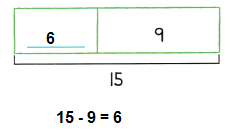FOR THE TEACHER • Discuss with children how this model can be used to represent an addition or a subtraction situation.
In the above model, subtraction situation can be discussed with children,
as total marble are 15 and he gave 9 marble to his friend, then 6 more marble left with him.

Math Talk
Mathematical Processes

To solve story problem we use subtraction.
In subtraction we subtract the desired marbles from the total to get the sum.

Model and Draw

A number sentence can be used to show a problem.
There were some girls and 4 boys at the playground. There were 9 children in all. How many girls were at the playground?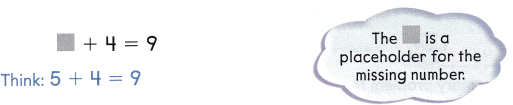So, there were __________ girls at the playground.
Explanation:
There were some girls and 4 boys at the playground.
There were 9 children in all.
Number of girls at the playground
5 + 4 = 9

Share and Show

Write a number sentence for the problem. Use a ☐ for the missing number. Then solve.

Question 1.
There were 14 ants on the sidewalk. Then 6 ants went into the grass. How many ants were still on the sidewalk?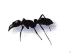__________ ants
8 ants
Explanation:
There were 14 ants on the sidewalk.
Then 6 ants went into the grass.
Number of ants still on the sidewalk
14 – 6 = 8

Question 2.
There were 7 big dogs and 4 little dogs at the park. How many dogs were at the park?__________ dogs
11 dogs
Explanation:
There were 7 big dogs and 4 little dogs at the park.
Number of dogs at the park
7 + 4 = 11

Problem Solving

Write a number sentence for the problem. Use a ☐ for the missing number. Then solve.

Question 3.
There were 13 girls flying kites. Some of the girls went home. Then there were 7 girls flying kites. How many girls went home?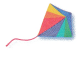__________ girls
6 girls
There were 13 girls flying kites.
Some of the girls went home.
Then there were 7 girls flying kites.
Number of girls went home
13 – 7 = 6

Question 4.
There are 18 boys at the field. 9 of the boys are playing soccer. How many boys are not playing soccer?___________ boys
9 boys
Explanation:
There are 18 boys at the field.
9 of the boys are playing soccer.
Number of boys not playing soccer
18 – 9 = 9

Solve. Write or draw to explain.

Question 5.
H.O.T. There were some ducks in a pond. Four more ducks joined them. Then there were 12 ducks in the pond. How many ducks were in the pond at first?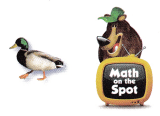_________ ducks
8 ducks
Explanation:
There were some ducks in a pond.
Four more ducks joined them.
Then there were 12 ducks in the pond.
Number of ducks in the pond at first
12 – 4 = 8

Question 6.
Multi-Step Matthew found 13 acorns. He traded 4 of his acorns for 7 acorns that Greg had. How many acorns does Matthew have now?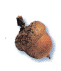__________ acorns
2 acorns
Explanation:
Matthew found 13 acorns.
He traded 4 of his acorns for 7 acorns that Greg had.
Number of  acorns  Matthew have now
13 – (4+7) = 13 – 11= 2

Question 7.
Apply Kent and Clark are playing a game. Clark has 11 points. That is 5 points more than Kent has. How many points does Kent have?
(A) 7
(B) 16
(C) 6
Option (C)
Explanation:
Clark has 11 points.
That is 5 points more than Kent has.
Number points Kent have
11 – 5 = 6

Question 8.
Multi-Step 4 children are playing soccer, 6 children are playing basketball and 3 children are playing catch. How many children are playing games?
(A) 10
(B) 13
(C) 9
Option ( B)
Explanation:
4 children are playing soccer,
6 children are playing basketball and
3 children are playing catch.
Number of children playing games
4 + 6 + 3 = 13

Question 9.
There are 5 children and 9 adults watching a game. How many people are watching the game?
(A) 12
(B) 16
(C) 14
Option ( C)
Explanation:
There are 5 children and
Number of people watching the game
5 + 9 =14

Question 10.
TEXAS Test Prep Jon counted 12 butterflies. Tessa counted 7 butterflies. How many fewer butterflies did Tessa count than Jon?(A) 7
(B) 5
(C) 19
Option (B)
Explanation:
Jon counted 12 butterflies.
Tessa counted 7 butterflies.
Number of fewer butterflies did Tessa count than Jon
12 – 7 = 5

After solving the above problems the child can do better in addition and subtractions equations.

### Texas Go Math Grade 2 Lesson 5.5 Homework and Practice Answer Key

Write a number sentence for the problem. Use a ☐ for the missing number. Then solve.

Question 1.
There were 11 boys riding bikes. Some of the boys left to drink water. Then there were 5 boys riding bikes. How many boys went to drink water?
_________ boys
6 boys
Explanation:
There were 11 boys riding bikes.
Some of the boys left to drink water.
Then there were 5 boys riding bikes.
Number of boys went to drink water
11 – 5 = 6

Question 2.
There are 7 runners at the track. Then walkers came to the track. Now there are 14 people at the track. How many walkers came to the track?
___________ walkers
7 walkers
Explanation:
There are 7 runners at the track.
Then walkers came to the track.
Now there are 14 people at the track.
Number of walkers came to the track
14 – 7 = 7
Problem Solving

Solve. Write or draw to explain.

Question 3.
There were some apples on the ground. Five more apples fell to the ground. Then there were 14 apples on the ground. How-many apples were there on the ground first?
_________ apples
9 apples
Explanation:
There were some apples on the ground.
Five more apples fell to the ground.
Then there were 14 apples on the ground.
Number of apples were there on the ground first
14 – 5 = 9

Question 4.
Multi-Step Kayla found 10 coins in a jar. She gave 3 coins to her sister. Then Kayla found 6 more coins on a table. How many coins does Kayla have now?
_____________ coins
13 coins
Explanation:
Kayla found 10 coins in a jar.
She gave 3 coins to her sister.
Then Kayla found 6 more coins on a table.
Number of coins does Kayla have now
10 – 3 = 7
7 + 6 = 13

Lesson Check

Question 5.
Jan counted 14 water bottles and Rita counted 6 water bottles. How many fewer water bottles did Rita count than Jan?
(A) 8
(B) 7
(C) 20
Option(A)
Explanation:
Jan counted 14 water bottles and
Rita counted 6 water bottles.
Number of  fewer water bottles did Rita count than Jan
14 – 6 = 8

Question 6.
There are 7 children and 6 adults at the dinner table. How many people are having dinner?
(A) 7
(B) 13
(C) 12
Option (B)
There are 7 children and 6 adults at the dinner table.
Number of people having dinner
7 + 6 = 13

Question 7.
Elliot and David are playing basketball. David won the game with 15 points. That was 7 points more than Elliot had. How many points did Elliot have?
(A) 8
(B) 1
(C) 9
Option (A)
Explanation:
David won the game with 15 points.
That was 7 points more than Elliot had.
Number of points Elliot have
15 – 7 = 8

Question 8.
Multi-Step At the beach, 7 children are swimming and 5 children are playing in the sand. There are 4 other children who are reading. How many children are at beach?
(A) 12
(B) 16
(C) 9